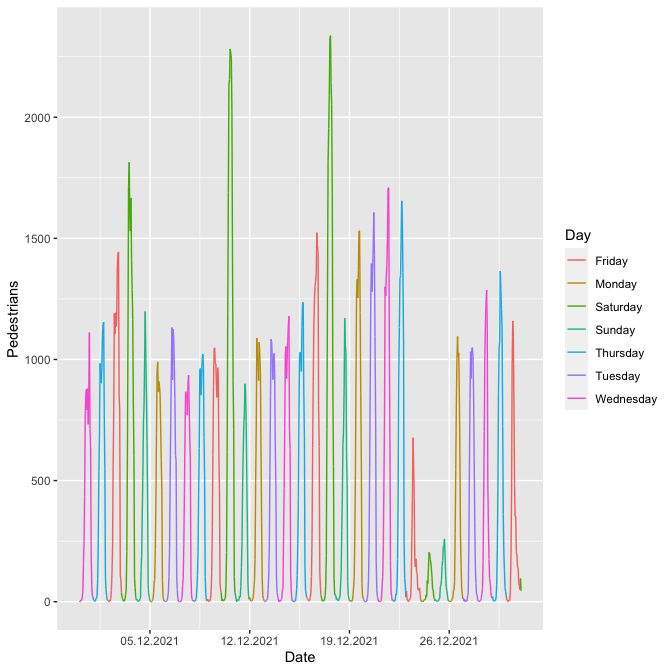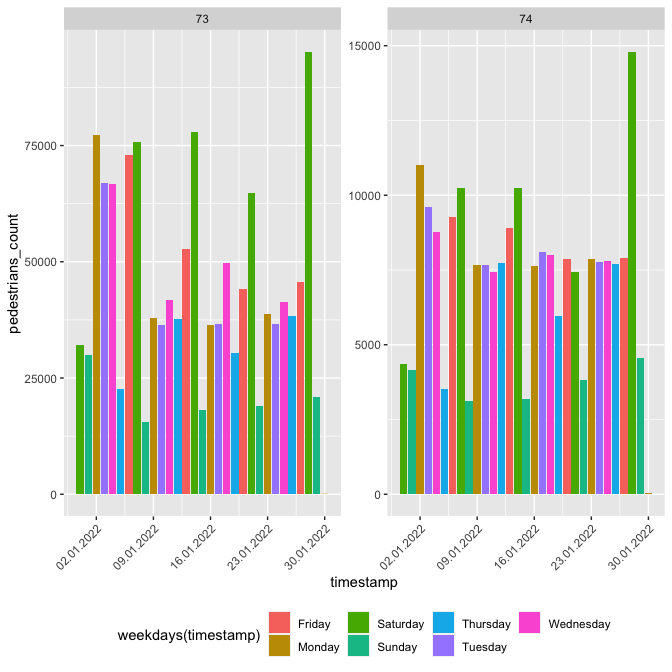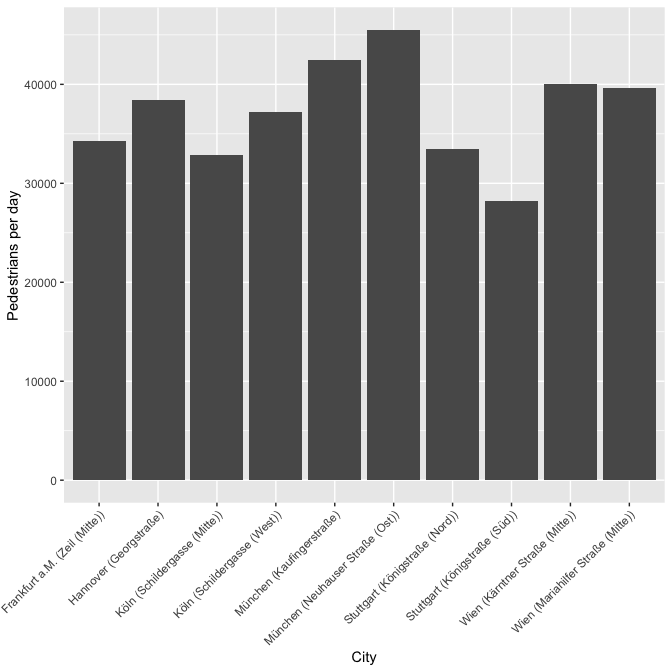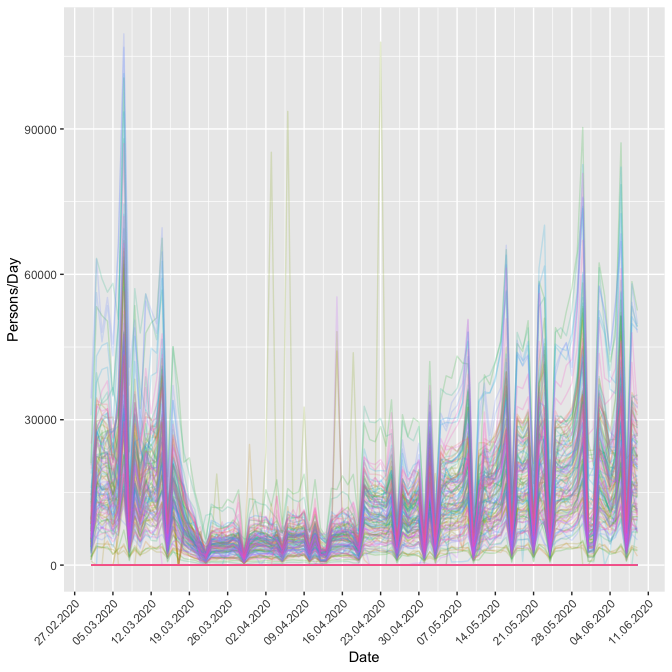# 2 Installation

Until now the package is not on CRAN but you can download it via GitHub with the following command:

# 3 API Keys

To use this package, you will first need to get a hystreet API key. To do so, you first need to set up an account on https://hystreet.com/. After that you can request an API key via e-mail. Once your request has been granted, you will find you key in your hystreet account profile.

Now you have three options:

1. Once you have your key, save it as an environment variable for the current session by running the following:
Sys.setenv(HYSTREET_API_TOKEN = "PASTE YOUR API TOKEN HERE")
1. Alternatively, you can set it permanently with the help of usethis::edit_r_environ() by adding the line to your .Renviron:
HYSTREET_API_TOKEN = PASTE YOUR API TOKEN HERE
1. If you don’t want to save it here, you can input it in each function using the API_token parameter.

# 4 Usage

Function name Description Example
get_hystreet_stats() request common statistics about the hystreet project get_hystreet_stats()
get_hystreet_locations() request all available locations get_hystreet_locations()
get_hystreet_station_data() request data from a stations get_hystreet_station_data(71)
set_hystreet_token() set your API token set_hystreet_token(123456789)

The function ‘get_hystreet_stats()’ summaries the number of available stations and the sum of all counted pedestrians.

library(hystReet)

stats <- get_hystreet_stats()

## 4.2 Request all stations

The function ‘get_hystreet_locations()’ requests all available stations of the project.

locations <- get_hystreet_locations()
id name city
360 Brüderstraße (Mitte) Soest
308 Leipziger Straße (West) Halle (Saale)
76 Königstraße (Mitte) Stuttgart
351 Johann-Philipp-Straße Trier
309 Hochstraße (Nord) Krefeld
140 Fleischstraße (Nord) Trier
55 Flinger Straße (Ost) Düsseldorf
108 Große Straße (Mitte) Osnabrück
348 Holstenstraße (Nord) Kiel

## 4.3 Request data from a specific station

The (probably) most interesting function is ‘get_hystreet_station_data()’. With the hystreetID it is possible to request a specific station. By default, all the data from the current day are received. With the ‘query’ argument it is possible to set the received data more precise:

• from: datetime of earliest measurement (default: today 00:00:00:): e.g. “2021-10-01 12:00:00” or “2021-10-01”
• to : datetime of latest measurement (default: today 23:59:59): e.g. “2021-12-01 12:00:00” or “2021-12-01”
• resolution: Resolution for the measurement grouping (default: hour): “day”, “hour”, “month”, “week”
location_71 <- get_hystreet_station_data(
hystreetId = 71,
query = list(from = "2021-12-01", to = "2022-01-01", resolution = "day"))

# 5 Some ideas to visualise the data

Let´s see if we can see the most frequent days before Christmas … I think it could be Saturday ;-). Also nice to see the 25th and 26th of December … holidays in Germany :-).

location_71 <- get_hystreet_station_data(
hystreetId = 71,
query = list(from = "2021-12-01", to = "2022-01-01", resolution = "hour"))
ggplot(location_71$measurements, aes(x = timestamp, y = pedestrians_count, colour = weekdays(timestamp))) + geom_path(group = 1) + scale_x_datetime(date_breaks = "7 days") + scale_x_datetime(labels = date_format("%d.%m.%Y")) + labs(x = "Date", y = "Pedestrians", colour = "Day") ## Scale for x is already present. ## Adding another scale for x, which will replace the existing scale.## 5.1 Compare different stations Now let´s compare different stations: 1. Load the data location_73 <- get_hystreet_station_data( hystreetId = 73, query = list(from = "2022-01-01", to = "2022-01-31", resolution = "day"))$measurements %>%
select(pedestrians_count, timestamp) %>%
mutate(station = 73)

location_74 <- get_hystreet_station_data(
hystreetId = 74,
query = list(from = "2022-01-01", to = "2019-01-22", resolution = "day"))$measurements %>% select(pedestrians_count, timestamp) %>% mutate(station = 74) data_73_74 <- bind_rows(location_73, location_74) ggplot(data_73_74, aes(x = timestamp, y = pedestrians_count, fill = weekdays(timestamp))) + geom_bar(stat = "identity") + scale_x_datetime(labels = date_format("%d.%m.%Y")) + facet_wrap(~station, scales = "free_y") + theme(legend.position = "bottom", axis.text.x = element_text(angle = 45, hjust = 1))## 5.2 Highest ratio (pedestrians/day) Now a little bit of big data analysis. Let´s find the station with the highest pedestrians per day ratio: hystreet_ids <- get_hystreet_locations() all_data <- lapply(hystreet_ids[,"id"], function(ID){ temp <- get_hystreet_station_data( hystreetId = ID, query = list(from = "2021-01-01", to = "2021-12-31", resolution = "day")) lifetime_count <- temp$statistics$timerange_count days_counted <- as.integer(ymd(temp$metadata$measured_to) - ymd(temp$metadata$measured_from)) return(data.frame( id = ID, station = paste0(temp$city, " (",temp$name,")"), ratio = lifetime_count/days_counted)) }) ratio <- bind_rows(all_data) What stations have the highest ratio? ratio %>% top_n(5, ratio) %>% arrange(desc(ratio)) ## id station ratio ## 1 73 München (Neuhauser Straße (Ost)) 45510.15 ## 2 165 München (Kaufingerstraße) 42458.42 ## 3 305 Wien (Kärntner Straße (Mitte)) 40067.34 ## 4 306 Wien (Mariahilfer Straße (Mitte)) 39642.14 ## 5 63 Hannover (Georgstraße) 38442.91 Now let´s visualise the top 10 cities: ggplot(ratio %>% top_n(10,ratio), aes(station, ratio)) + geom_bar(stat = "identity") + labs(x = "City", y = "Pedestrians per day") + theme(legend.position = "bottom", axis.text.x = element_text(angle = 45, hjust = 1))## 5.3 Corona effects The Hystreet-API is a great source of analysing the social effects of the Corona pandemic in 2020. Let´s collect all german stations since March 2020 and analyse the pedestrian count until 10th June 2020. data <- lapply(hystreet_ids[,"id"], function(ID){ temp <- get_hystreet_station_data( hystreetId = ID, query = list(from = "2020-03-01", to = "2020-06-10", resolution = "day") ) return(data.frame( name = temp$name,
city = temp$city, timestamp = format(as.POSIXct(temp$measurements$timestamp), "%Y-%m-%d"), pedestrians_count = temp$measurements$pedestrians_count, legend = paste(temp$city, temp\$name, sep = " - ")
))

})

corona_data_all <- bind_rows(data)
corona_data_all %>%
ggplot(aes(ymd(timestamp), pedestrians_count, colour = legend)) +
geom_line(alpha = 0.2) +
scale_x_date(labels = date_format("%d.%m.%Y"),
breaks = date_breaks("7 days")
) +
theme(legend.position = "none",
legend.title = element_text("Legende"),
axis.text.x = element_text(angle = 45, hjust = 1)) +
labs(x = "Date",
y = "Persons/Day")